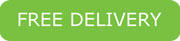#### Calculate Materials

You can use these calculators [below] to determine approximately how many cubic yards of material you will need for landscape projects.

[If you are having trouble with our calculators,  please try Internet Explorer or Chrome]

To use this calculator, simply insert your dimensions in the designated input fields separatley.

then click “Calculate” – the calculator will do the rest.

Remember: A calculator is only as good as its measurer.

Total

##### To calculate thickness:

The size of your material will determine how thick you should place your material.

3/8″ = 1″ to 2″
3/4″ = 2″ to 3″
1 1/2″ = 3″ to 31/2″

Total

#### Weight Per Yard

Looking to pick up your own materials and want to know the weight?

The chart below will give you a rough estimate of the weight.

Due to the moisture content of materials, the products’ weight will vary.

We can load in ¼ yard increments: \$10 minimum for quarter yard

Total

To calculate yardage:

Length x Width x Depth = Cubic Feet

Divide by 27 (27 cubic feet in a yard) = Cubic Yards

#### Depth Conversion to Feet

 1" = .08 7" = .58 2" = .18 8" = .67 3" = .25 9" = .75 4" = .33 10" = .83 5" = .41 11" = .92 6" = .50 12" = 1.0
 PRODUCT WEIGHT lbs/yard Barks 500-600 Soils 1340-1600 Compost 1400-1600 Sands 2600-2850 Decomposed Granite 2600-2850 Cobbles 2600-2850 Rocks 2600-2850free delivery
for 15 yards of one item
in City Limits